Students can Download Computer Science Chapter 2 Data Abstraction Questions and Answers, Notes Pdf, Samacheer Kalvi 12th Computer Science Book Solutions Guide Pdf helps you to revise the complete Tamilnadu State Board New Syllabus and score more marks in your examinations.

## Tamilnadu Samacheer Kalvi 12th Computer Science Solutions Chapter 2 Data Abstraction

### Samacheer Kalvi 12th Computer Science Data Abstraction Text Book Back Questions and Answers

PART – I

Question 1.
Which of the following functions that build the abstract data type?
(a) Constructors
(b) Destructors
(c) Recursive
(d) Nested
(a) Constructors

Question 2.
Which of the following functions that retrieve information from the data type?
(a) Constructors
(b) Selectors
(c) Recursive
(d) Nested
(b) SelectorsQuestion 3.
The data structure which is a mutable ordered sequence of elements is called ………………………
(a) Built in
(b) List
(c) Tuple
(d) Derived data
(b) List

Question 4.
A sequence of immutable objects is called ………………………
(a) Built in
(b) List
(c) Tuple
(d) Derived data
(c) Tuple

Question 5.
The data type whose representation is known are called ………………………
(a) Built in data type
(b) Derived data type
(c) Concrete data type
(d) Abstract data type
(c) Concrete data typeQuestion 6.
The data type whose representation is unknown are called ………………………
(a) Built in data type
(b) Derived data type
(c) Concrete data type
(d) Abstract datatype
(d) Abstract datatype

Question 7.
Which of the following is a compound structure?
(a) Pair
(b) Triplet
(c) Single
(a) PairQuestion 8.
Bundling two values together into one can be considered as ………………………
(a) Pair
(b) Triplet
(c) Single
(a) Pair

Question 9.
Which of the following allow to name the various parts of a multi – item object?
(a) Tuples
(b) Lists
(c) Classes
(c) ClassesQuestion 10.
Which of the following is constructed by placing expressions within square brackets?
(a) Tuples
(b) Lists
(c) Classes
(b) Lists

PART – II

Question 1.
What is abstract data type?
Abstract Data type (ADT) is a type (or class) for objects whose behavior is defined by a set of value and a set of operations. The definition of ADT only mentions what operations are to be performed but not how these operations will be implemented.Question 2.
Differentiate constructors and selectors?
Constructors are functions that build the abstract data type. Selectors are functions that retrieve information from the data type.
To create a city object, you’d use a function like
city = makecity (name, lat, Ion)
To extract the information of a city object, you would use functions like
getname (city)Question 3.
What is a Pair? Give an example?
Pair is a compound structure which is made up of list or Tuple.
lst[(0, 10), (1, 20)] -where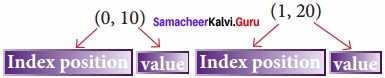Any way of bundling two values together into one can be considered as a pair. Lists are a common method to do so. Therefore List can be called as Pairs.

Question 4.
What is a List? Give an example?
List is constructed by placing expressions within square brackets separated by commas. Example for List is [10, 20].Question 5.
What is a Tuple? Give an example?
A tuple is a comma-separated sequence of values surrounded with parentheses. Tuple is similar to a list. The difference between the two is that you cannot change the elements of a tuple once it is assigned whereas in a list, elements can be changed.
Example colour = (‘red’, ‘blue’, ‘Green’)

PART – III

Question 1.
Differentiate Concrete data type and abstract datatype?
Concrete data type:

1. A concrete data type is a data type whose representation is known.
2. Concrete data types or structures (CDT’s) are direct implementations of a relatively simple concept.

Abstract data type:

1. Abstract data type the representation of a data type is unknown.
2. Abstract Data Types (ADT’s) offer a high level view (and use) of a concept independent of its implementation.Question 2.
Which strategy is used for program designing? Define that Strategy?
We are using here a powerful strategy for designing programs: ‘wishful thinking’.
Wishful Thinking is the formation of beliefs and making decisions according to what might be pleasing to imagine instead of by . appealing to reality.

Question 3.
Identify Which of the following are constructors and selectors?
(a) N1 = number ( ) – constructors
(b) Accetnum (n1) – selectors
(c) Displaynum (n1) – selectors
(d) eval (a/b) – selectors
(e) x, y = makeslope(m), makeslope (n) – constructors
(f) display O – selectorsQuestion 4.
What are the different ways to access the elements of a list. Give example?
List is constructed by placing expressions within square brackets separated by commas. Example for List is [10, 20].
The elements of a list can be accessed in two ways. The first way is via our familiar method of multiple assignment, which unpacks a list into its elements and binds each element to a different name.
1st: = [10, 20]
x, y: = 1st
In the above example x will become 10 and y will become 20.
A second method for accessing the elements in a list is by the element selection operator, also expressed using square brackets. Unlike a list literal, a square – brackets expression directly following another expression does not evaluate to a list value, but instead selects an element from the value of the preceding expression.
1st 
10
1st 
20Question 5.
Identify Which of the following are List, Tuple and class?
(a) arr [1, 2, 34]
(b) arr (1, 2, 34)
(c) student [rno, name, mark]
(d) day = (‘sun’, ‘mon’, ‘tue’, ‘wed’)
(e) x= [2, 5, 6.5, [5,6], 8.2]
(f) employee [eno, ename, esal, eaddress]
List: (a) arr [1, 2, 34]
(e) x= [2, 5, 6.5, [5,6], 8.2]
Tuple: (b) arr (1, 2, 34)
(d) day = (‘sun’, ‘mon’, ‘tue’, ‘wed’)
Class: (c) student [mo, name, mark]
(f) employee [eno, ename, esal, eaddress]

PART – IV

Question 1.
How will you facilitate data abstraction. Explain it with suitable example?
The definition of ADT only mentions what operations are to be performed but not how these operations will be implemented. It does not specify how data will be organized in memory and what algorithms will be used for implementing the operations. It is called “abstract” because it gives an implementation independent view. The process of providing only the essentials and hiding the details is known as abstraction.
To facilitate data abstraction, you will need to create two types of functions.

constructors and selectors:
Constructors are functions that build the abstract data type. Selectors are functions that retrieve information from the data type.
To create a city object, you’d use a function like city = makecity (name, lat, Ion)
To extract the information of a city object, you would use functions like

1. getname(city)
2. getlat(city)
3. getlon(city)

In the above pseudo code the function which creates the object of the city is the constructor, city = makecity (name, lat, Ion)
Here makecity (name, lat, Ion) is the constructor which creates the object city.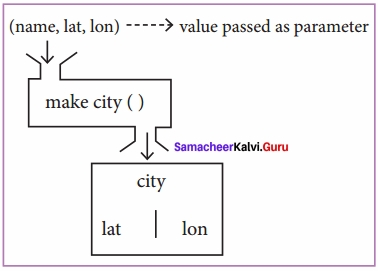Selectors are nothing but the functions that retrieve information from the data type. Therefore in the above code

1. getname(city)
2. getlat(city)
3. getlon(city)

are the selectors because these functions extract the information of the city object.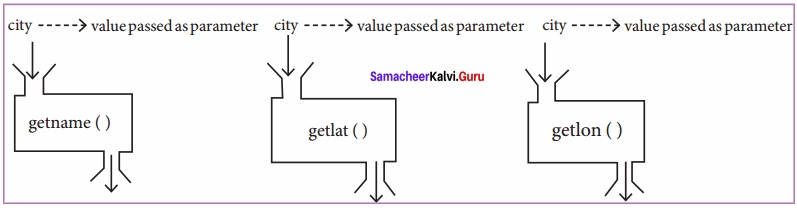Data abstraction is supported by defining an abstract data type (ADT), which is a collection of constructors and selectors. Constructors create an object, bundling together different pieces of information, while selectors extract individual pieces of information from the object.Question 2.
What is a List? Why List can be called as Pairs. Explain with suitable example?
List is constructed by placing expressions within square brackets separated by commas. Example for List is [10, 20],
The elements of a list can be accessed in two ways. The first way is via our familiar method of multiple assignment, which unpacks a list into its elements and binds each element to a different name.
1st: = [10, 20]
x, y: = 1st
In the above example x will become 10 and y will become 20.
A second method for accessing the elements in a list is by the element selection operator, also expressed using square brackets. Unlike a list literal, a square – brackets expression directly following another expression does not evaluate to a list value, but instead selects an element from the value of the preceding expression.
1st 
10
1st 
20
In both the example mentioned above mathematically we can represent list similar to a set.
1st [(0, 10), (1, 20)] – where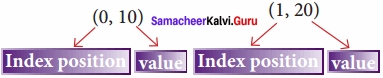Any way of bundling two values together into one can be considered as a pair. Lists are a common method to do so. Therefore List can be called as Pairs.Question 3.
How will you access the multi – item. Explain with example?
List allow data abstraction in that you can give a name to a set of memory cells. For instance, in the game Mastermind, you must keep track of a list of four colors that the player guesses. Instead of using four separate variables (color 1, color2, color3, and color4) you can use a single variable ‘Predict’, e.g.,
Predict = [‘red’, ‘blue’, ‘green’, ’green’]
What lists do not allow us to do is name the various parts of a multi- item object. In the case of a Predict, you don’t really need to name the parts:
using an index to get to each color suffices.
But in the case of something more complex, like a person, we have a multi – item object where each ‘item’ is a named thing: the firstName, the last Name, the id, and the email. One could use a list to represent a person.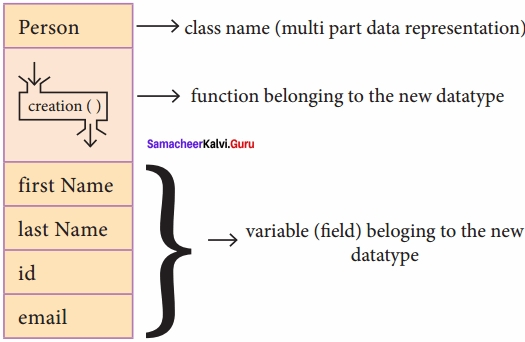but such a representation doesn’t explicitly specify what each part represents.
For this problem instead of using a list, you can use the structure constmct (In OOP languages it’s called class construct) to represent multi-part objects where each part is named (given a name). Consider the following pseudo code:
class Person:
creation( )
firstName: = “”
lastName: = ” ”
id: = ” ”
email : = “”
The new data type Person is pictorially represented as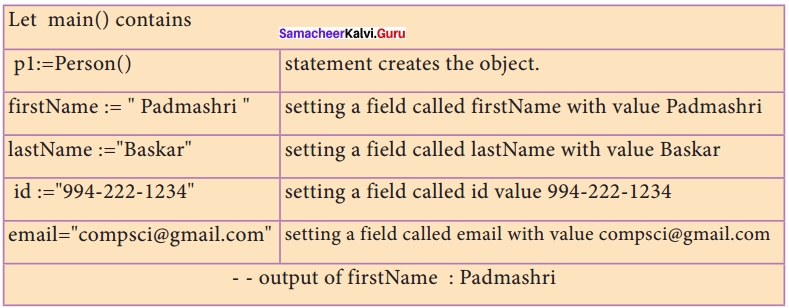The class (structure) constmct defines the form for multi – part objects that represent a person. Its defintion adds a new data type, in this case a type named Person. Once defined, we can create new variables (instances) of the type. In this example Person is referred to as a class or a type, while p1 is referred to as an object or an instance. You can think of class Person as a cookie cutter, and p1 as a particular cookie. Using the cookie cutter you can make many cookies. Same way using class you can create many objects of that type.

### Samacheer kalvi 12th Computer Science Data Abstraction Additional Questions and Answers

PART – 1

Question 1.
How many types of functions are needed to facilitate abstraction?
(a) 1
(b) 2
(c) 3
(d) 4
(b) 2Question 2.
(b) Application Developing Tool
(c) Abstract data types
(c) Abstract data types

Question 3.
The Splitting of program into many modules are called as ……………………………
(a) Modularity
(b) Structures
(c) Classes
(d) List
(a) ModularityQuestion 4.
……………………………. are the representation for ADT.
(a) List
(b) Classes
(c) Int
(d) Float
(b) Classes

Question 5.
(a) Single
(b) Double
(c) Multiple
(d) Both a and b
(d) Both a and bQuestion 6.
The process of providing only the essentials and hiding the details is known as …………………………..
(a) Modularity
(b) Structure
(c) Tuple
(d) Abstraction
(d) Abstraction

Question 7.
Identify the constructor from the following
(a) City = makecity(name, lat, lon)
(b) getname(city)
(c) getlat(city)
(d) getlon(city)
(a) City = makecity(name, lat, lon)Question 8.
: = is called as …………………………..
(a) Assigned as
(b) Becomes
(c) Both a and b
(d) None of these
(c) Both a and b

Question 9.
In list 1st [(0, 10), (1, 20)] – 0 and 1 represents …………………………..
(a) Value
(b) Index
(c) List identifier
(d) Tuple
(b) IndexQuestion 10.
How many ways of representing pair data type are there?
(a) 1
(b) 2
(c) 3
(d) 4
(b) 2

Question 11.
nums  represent that you are accessing ………………………….. element.
(a) 0
(b) 1
(c) 2
(d) 3
(b) 1Question 12.
nums  indicate that we are accessing ………………………….. element.
(a) 0
(b) 1
(c) 2
(d) many
(c) 2

Question 13.
How many objects can be created from a class?
(a) 0
(b) 1
(c) 2
(d) many
(d) many

PART – II

Question 1.
What are the two parts of a program?
The two parts of a program are, the part that operates on abstract data and the part that defines a concrete representation.Question 2.
Give the pseudo code to represent a rational number as a pair of two integers?
You can now represent a rational number as a pair of two integers in pseudo code: a numerator and a denominator.
rational (n, d):
return [n, d]
numer (x):
return x 
denom (x):
return x 

Question 3.
What are the two ways of representing the pair data type?
Two ways of representing the pair data type. The first way is using List construct and the second way to implement pairs is with the tuple construct.Question 4.
Differentiate tuple and list?
List:
In List square bracket is used.

Tuple:
In Tuple parenthesis is used.

Question 5.
Give an example for representation of Tuple as a pair?
Representation of Tuple as a Pair
nums : = (1, 2)
nums 
1
nums 
2Question 6.
Define class?